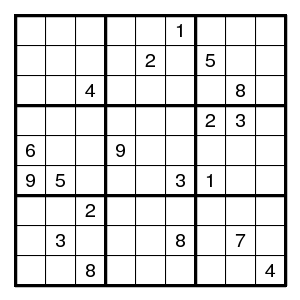Prev Up Next

# Alternating digits

A reasoning can follow a chain of positions on the sudoku grid. There are many kinds of chains. Here an example of the use of the simplest type, namely that where the links are double pairs.

Consider the following puzzle.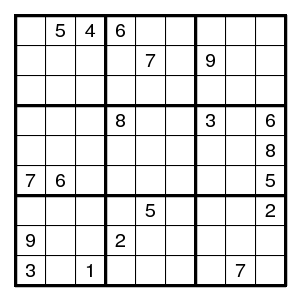First some routine work.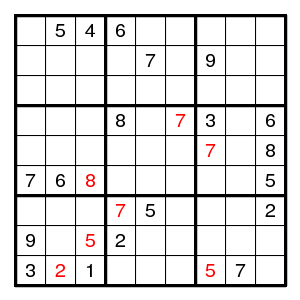There is only one place for digit 5 in row 4 and for digit 9 in column 9.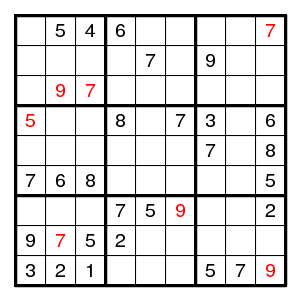There is only one place for digit 3 in rows 7,1,3,8,6. For digit 1 in row 7 and column 9.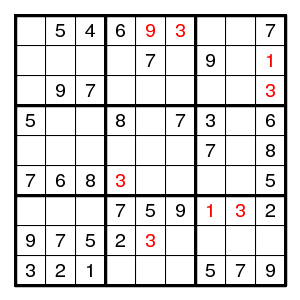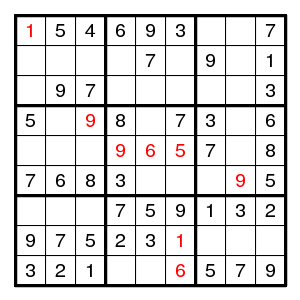There is only one possibility for (row 7, column3).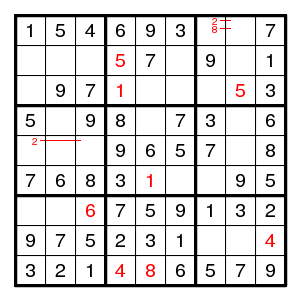So far all was easy, but what next? Let us write all possibilities in the open squares.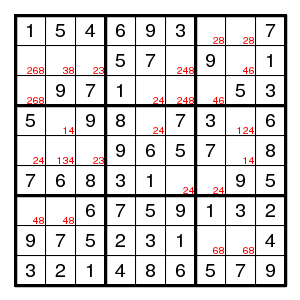No very obvious conclusions. After staring at this for a while, look: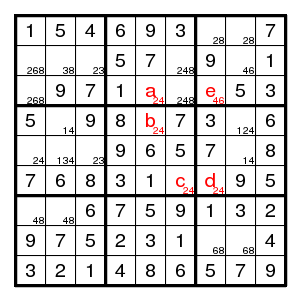In the chain a,b,c,d the values alternate between 2 and 4, so that either a or d is 4. But then e cannot be 4 and must be 6. Further completion is completely uneventful.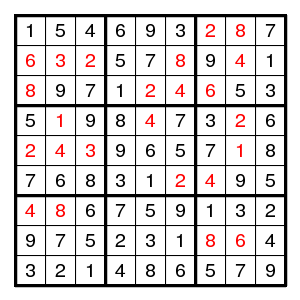Terminology A pair like a-d above is sometimes called a 'remote pair'.

## Exercise

Try the following. It uses everything seen so far.